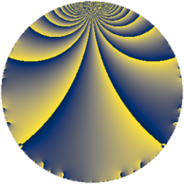Properties

 Label 6384.2.a.bsLevel $6384$ Weight $2$ Character orbit 6384.a Self dual yes Analytic conductor $50.976$ Analytic rank $1$ Dimension $2$ CM no Inner twists $1$

Related objects

Newspace parameters

 Level: $$N$$ $$=$$ $$6384 = 2^{4} \cdot 3 \cdot 7 \cdot 19$$ Weight: $$k$$ $$=$$ $$2$$ Character orbit: $$[\chi]$$ $$=$$ 6384.a (trivial)

Newform invariants

 Self dual: yes Analytic conductor: $$50.9764966504$$ Analytic rank: $$1$$ Dimension: $$2$$ Coefficient field: $$\Q(\sqrt{3})$$ Defining polynomial: $$x^{2} - 3$$ Coefficient ring: $$\Z[a_1, \ldots, a_{5}]$$ Coefficient ring index: $$1$$ Twist minimal: no (minimal twist has level 3192) Fricke sign: $$1$$ Sato-Tate group: $\mathrm{SU}(2)$

$q$-expansion

Coefficients of the $$q$$-expansion are expressed in terms of $$\beta = \sqrt{3}$$. We also show the integral $$q$$-expansion of the trace form.

 $$f(q)$$ $$=$$ $$q + q^{3} + ( 1 + \beta ) q^{5} - q^{7} + q^{9} +O(q^{10})$$ $$q + q^{3} + ( 1 + \beta ) q^{5} - q^{7} + q^{9} + ( -2 - 2 \beta ) q^{13} + ( 1 + \beta ) q^{15} + ( -3 + \beta ) q^{17} + q^{19} - q^{21} + ( -1 + 2 \beta ) q^{25} + q^{27} + ( -1 - 5 \beta ) q^{29} -2 q^{31} + ( -1 - \beta ) q^{35} -10 q^{37} + ( -2 - 2 \beta ) q^{39} + ( -2 - 4 \beta ) q^{41} + ( 2 - 4 \beta ) q^{43} + ( 1 + \beta ) q^{45} + ( 3 + 5 \beta ) q^{47} + q^{49} + ( -3 + \beta ) q^{51} + ( -5 - \beta ) q^{53} + q^{57} -8 q^{59} -2 q^{61} - q^{63} + ( -8 - 4 \beta ) q^{65} + ( 10 + 2 \beta ) q^{67} + ( -3 - \beta ) q^{71} + 6 \beta q^{73} + ( -1 + 2 \beta ) q^{75} -4 q^{79} + q^{81} + ( -5 - 3 \beta ) q^{83} -2 \beta q^{85} + ( -1 - 5 \beta ) q^{87} + ( -2 + 4 \beta ) q^{89} + ( 2 + 2 \beta ) q^{91} -2 q^{93} + ( 1 + \beta ) q^{95} + 2 q^{97} +O(q^{100})$$ $$\operatorname{Tr}(f)(q)$$ $$=$$ $$2 q + 2 q^{3} + 2 q^{5} - 2 q^{7} + 2 q^{9} + O(q^{10})$$ $$2 q + 2 q^{3} + 2 q^{5} - 2 q^{7} + 2 q^{9} - 4 q^{13} + 2 q^{15} - 6 q^{17} + 2 q^{19} - 2 q^{21} - 2 q^{25} + 2 q^{27} - 2 q^{29} - 4 q^{31} - 2 q^{35} - 20 q^{37} - 4 q^{39} - 4 q^{41} + 4 q^{43} + 2 q^{45} + 6 q^{47} + 2 q^{49} - 6 q^{51} - 10 q^{53} + 2 q^{57} - 16 q^{59} - 4 q^{61} - 2 q^{63} - 16 q^{65} + 20 q^{67} - 6 q^{71} - 2 q^{75} - 8 q^{79} + 2 q^{81} - 10 q^{83} - 2 q^{87} - 4 q^{89} + 4 q^{91} - 4 q^{93} + 2 q^{95} + 4 q^{97} + O(q^{100})$$

Embeddings

For each embedding $$\iota_m$$ of the coefficient field, the values $$\iota_m(a_n)$$ are shown below.

For more information on an embedded modular form you can click on its label.

Label $$\iota_m(\nu)$$ $$a_{2}$$ $$a_{3}$$ $$a_{4}$$ $$a_{5}$$ $$a_{6}$$ $$a_{7}$$ $$a_{8}$$ $$a_{9}$$ $$a_{10}$$
1.1
 −1.73205 1.73205
0 1.00000 0 −0.732051 0 −1.00000 0 1.00000 0
1.2 0 1.00000 0 2.73205 0 −1.00000 0 1.00000 0
 $$n$$: e.g. 2-40 or 990-1000 Significant digits: Format: Complex embeddings Normalized embeddings Satake parameters Satake angles

Atkin-Lehner signs

$$p$$ Sign
$$2$$ $$1$$
$$3$$ $$-1$$
$$7$$ $$1$$
$$19$$ $$-1$$

Inner twists

This newform does not admit any (nontrivial) inner twists.

Twists

By twisting character orbit
Char Parity Ord Mult Type Twist Min Dim
1.a even 1 1 trivial 6384.2.a.bs 2
4.b odd 2 1 3192.2.a.q 2
12.b even 2 1 9576.2.a.bj 2

By twisted newform orbit
Twist Min Dim Char Parity Ord Mult Type
3192.2.a.q 2 4.b odd 2 1
6384.2.a.bs 2 1.a even 1 1 trivial
9576.2.a.bj 2 12.b even 2 1

Hecke kernels

This newform subspace can be constructed as the intersection of the kernels of the following linear operators acting on $$S_{2}^{\mathrm{new}}(\Gamma_0(6384))$$:

 $$T_{5}^{2} - 2 T_{5} - 2$$ $$T_{11}$$ $$T_{13}^{2} + 4 T_{13} - 8$$ $$T_{17}^{2} + 6 T_{17} + 6$$ $$T_{23}$$

Hecke characteristic polynomials

$p$ $F_p(T)$
$2$ $$T^{2}$$
$3$ $$( -1 + T )^{2}$$
$5$ $$-2 - 2 T + T^{2}$$
$7$ $$( 1 + T )^{2}$$
$11$ $$T^{2}$$
$13$ $$-8 + 4 T + T^{2}$$
$17$ $$6 + 6 T + T^{2}$$
$19$ $$( -1 + T )^{2}$$
$23$ $$T^{2}$$
$29$ $$-74 + 2 T + T^{2}$$
$31$ $$( 2 + T )^{2}$$
$37$ $$( 10 + T )^{2}$$
$41$ $$-44 + 4 T + T^{2}$$
$43$ $$-44 - 4 T + T^{2}$$
$47$ $$-66 - 6 T + T^{2}$$
$53$ $$22 + 10 T + T^{2}$$
$59$ $$( 8 + T )^{2}$$
$61$ $$( 2 + T )^{2}$$
$67$ $$88 - 20 T + T^{2}$$
$71$ $$6 + 6 T + T^{2}$$
$73$ $$-108 + T^{2}$$
$79$ $$( 4 + T )^{2}$$
$83$ $$-2 + 10 T + T^{2}$$
$89$ $$-44 + 4 T + T^{2}$$
$97$ $$( -2 + T )^{2}$$